# perfcurve

Receiver operating characteristic (ROC) curve or other performance curve for classifier output

## Syntax

``````[X,Y] = perfcurve(labels,scores,posclass)``````
``````[X,Y,T] = perfcurve(labels,scores,posclass)``````
``````[X,Y,T,AUC] = perfcurve(labels,scores,posclass)``````
``````[X,Y,T,AUC,OPTROCPT] = perfcurve(labels,scores,posclass)``````
``````[X,Y,T,AUC,OPTROCPT,SUBY] = perfcurve(labels,scores,posclass)``````
``````[X,Y,T,AUC,OPTROCPT,SUBY,SUBYNAMES] = perfcurve(labels,scores,posclass)``````
``[___] = perfcurve(labels,scores,posclass,Name,Value)``

## Description

example

``````[X,Y] = perfcurve(labels,scores,posclass)``` returns the `X` and `Y` coordinates of an ROC curve for a vector of classifier predictions, `scores`, given true class labels, `labels`, and the positive class label, `posclass`. You can visualize the performance curve using `plot(X,Y)`.```
``````[X,Y,T] = perfcurve(labels,scores,posclass)``` returns an array of thresholds on classifier scores for the computed values of `X` and `Y`.```

example

``````[X,Y,T,AUC] = perfcurve(labels,scores,posclass)``` returns the area under the curve for the computed values of `X` and `Y`. ```

example

``````[X,Y,T,AUC,OPTROCPT] = perfcurve(labels,scores,posclass)``` returns the optimal operating point of the ROC curve.```
``````[X,Y,T,AUC,OPTROCPT,SUBY] = perfcurve(labels,scores,posclass)``` returns the `Y` values for negative subclasses.```

example

``````[X,Y,T,AUC,OPTROCPT,SUBY,SUBYNAMES] = perfcurve(labels,scores,posclass)``` returns the negative class names.```
````[___] = perfcurve(labels,scores,posclass,Name,Value)` returns the coordinates of a ROC curve and any other output argument from the previous syntaxes, with additional options specified by one or more `Name,Value` pair arguments.For example, you can provide a list of negative classes, change the `X` or `Y` criterion, compute pointwise confidence bounds using cross validation or bootstrap, specify the misclassification cost, or compute the confidence bounds in parallel.```

## Examples

collapse all

`load fisheriris`

Use only the first two features as predictor variables. Define a binary classification problem by using only the measurements that correspond to the species versicolor and virginica.

`pred = meas(51:end,1:2);`

Define the binary response variable.

`resp = (1:100)'>50; % Versicolor = 0, virginica = 1`

Fit a logistic regression model.

`mdl = fitglm(pred,resp,'Distribution','binomial','Link','logit');`

Compute the ROC curve. Use the probability estimates from the logistic regression model as scores.

```scores = mdl.Fitted.Probability; [X,Y,T,AUC] = perfcurve(species(51:end,:),scores,'virginica');```

`perfcurve` stores the threshold values in the array `T`.

Display the area under the curve.

`AUC`
```AUC = 0.7918 ```

The area under the curve is 0.7918. The maximum AUC is 1, which corresponds to a perfect classifier. Larger AUC values indicate better classifier performance.

Plot the ROC curve.

```plot(X,Y) xlabel('False positive rate') ylabel('True positive rate') title('ROC for Classification by Logistic Regression')```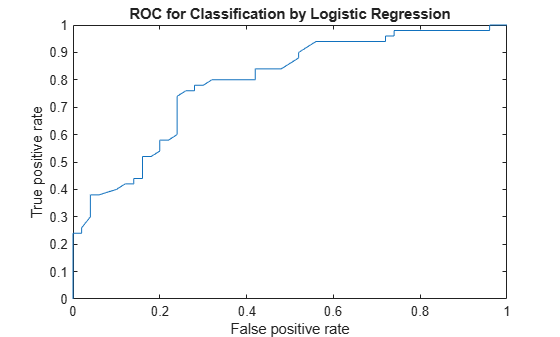`load ionosphere`

`X` is a 351x34 real-valued matrix of predictors. `Y` is a character array of class labels: `'b'` for bad radar returns and `'g'` for good radar returns.

Reformat the response to fit a logistic regression. Use the predictor variables 3 through 34.

```resp = strcmp(Y,'b'); % resp = 1, if Y = 'b', or 0 if Y = 'g' pred = X(:,3:34);```

```mdl = fitglm(pred,resp,'Distribution','binomial','Link','logit'); score_log = mdl.Fitted.Probability; % Probability estimates```

Compute the standard ROC curve using the probabilities for scores.

`[Xlog,Ylog,Tlog,AUClog] = perfcurve(resp,score_log,'true');`

Train an SVM classifier on the same sample data. Standardize the data.

`mdlSVM = fitcsvm(pred,resp,'Standardize',true);`

Compute the posterior probabilities (scores).

```mdlSVM = fitPosterior(mdlSVM); [~,score_svm] = resubPredict(mdlSVM);```

The second column of `score_svm` contains the posterior probabilities of bad radar returns.

Compute the standard ROC curve using the scores from the SVM model.

`[Xsvm,Ysvm,Tsvm,AUCsvm] = perfcurve(resp,score_svm(:,mdlSVM.ClassNames),'true');`

Fit a naive Bayes classifier on the same sample data.

`mdlNB = fitcnb(pred,resp);`

Compute the posterior probabilities (scores).

`[~,score_nb] = resubPredict(mdlNB);`

Compute the standard ROC curve using the scores from the naive Bayes classification.

`[Xnb,Ynb,Tnb,AUCnb] = perfcurve(resp,score_nb(:,mdlNB.ClassNames),'true');`

Plot the ROC curves on the same graph.

```plot(Xlog,Ylog) hold on plot(Xsvm,Ysvm) plot(Xnb,Ynb) legend('Logistic Regression','Support Vector Machines','Naive Bayes','Location','Best') xlabel('False positive rate'); ylabel('True positive rate'); title('ROC Curves for Logistic Regression, SVM, and Naive Bayes Classification') hold off```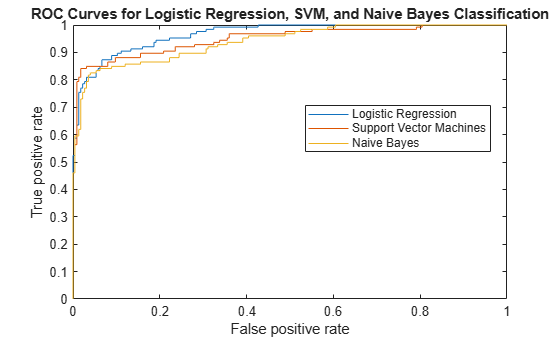Although SVM produces better ROC values for higher thresholds, logistic regression is usually better at distinguishing the bad radar returns from the good ones. The ROC curve for naive Bayes is generally lower than the other two ROC curves, which indicates worse in-sample performance than the other two classifier methods.

Compare the area under the curve for all three classifiers.

`AUClog`
```AUClog = 0.9659 ```
`AUCsvm`
```AUCsvm = 0.9489 ```
`AUCnb`
```AUCnb = 0.9393 ```

Logistic regression has the highest AUC measure for classification and naive Bayes has the lowest. This result suggests that logistic regression has better in-sample average performance for this sample data.

This example shows how to determine the better parameter value for a custom kernel function in a classifier using the ROC curves.

Generate a random set of points within the unit circle.

```rng(1); % For reproducibility n = 100; % Number of points per quadrant r1 = sqrt(rand(2*n,1)); % Random radii t1 = [pi/2*rand(n,1); (pi/2*rand(n,1)+pi)]; % Random angles for Q1 and Q3 X1 = [r1.*cos(t1) r1.*sin(t1)]; % Polar-to-Cartesian conversion r2 = sqrt(rand(2*n,1)); t2 = [pi/2*rand(n,1)+pi/2; (pi/2*rand(n,1)-pi/2)]; % Random angles for Q2 and Q4 X2 = [r2.*cos(t2) r2.*sin(t2)]; ```

Define the predictor variables. Label points in the first and third quadrants as belonging to the positive class, and those in the second and fourth quadrants in the negative class.

```pred = [X1; X2]; resp = ones(4*n,1); resp(2*n + 1:end) = -1; % Labels ```

Create the function `mysigmoid.m` , which accepts two matrices in the feature space as inputs, and transforms them into a Gram matrix using the sigmoid kernel.

```function G = mysigmoid(U,V) % Sigmoid kernel function with slope gamma and intercept c gamma = 1; c = -1; G = tanh(gamma*U*V' + c); end ```

Train an SVM classifier using the sigmoid kernel function. It is good practice to standardize the data.

```SVMModel1 = fitcsvm(pred,resp,'KernelFunction','mysigmoid',... 'Standardize',true); SVMModel1 = fitPosterior(SVMModel1); [~,scores1] = resubPredict(SVMModel1); ```

Set `gamma = 0.5` ; within `mysigmoid.m` and save as `mysigmoid2.m`. And, train an SVM classifier using the adjusted sigmoid kernel.

```function G = mysigmoid2(U,V) % Sigmoid kernel function with slope gamma and intercept c gamma = 0.5; c = -1; G = tanh(gamma*U*V' + c); end ```
```SVMModel2 = fitcsvm(pred,resp,'KernelFunction','mysigmoid2',... 'Standardize',true); SVMModel2 = fitPosterior(SVMModel2); [~,scores2] = resubPredict(SVMModel2); ```

Compute the ROC curves and the area under the curve (AUC) for both models.

```[x1,y1,~,auc1] = perfcurve(resp,scores1(:,2),1); [x2,y2,~,auc2] = perfcurve(resp,scores2(:,2),1); ```

Plot the ROC curves.

```plot(x1,y1) hold on plot(x2,y2) hold off legend('gamma = 1','gamma = 0.5','Location','SE'); xlabel('False positive rate'); ylabel('True positive rate'); title('ROC for classification by SVM'); ```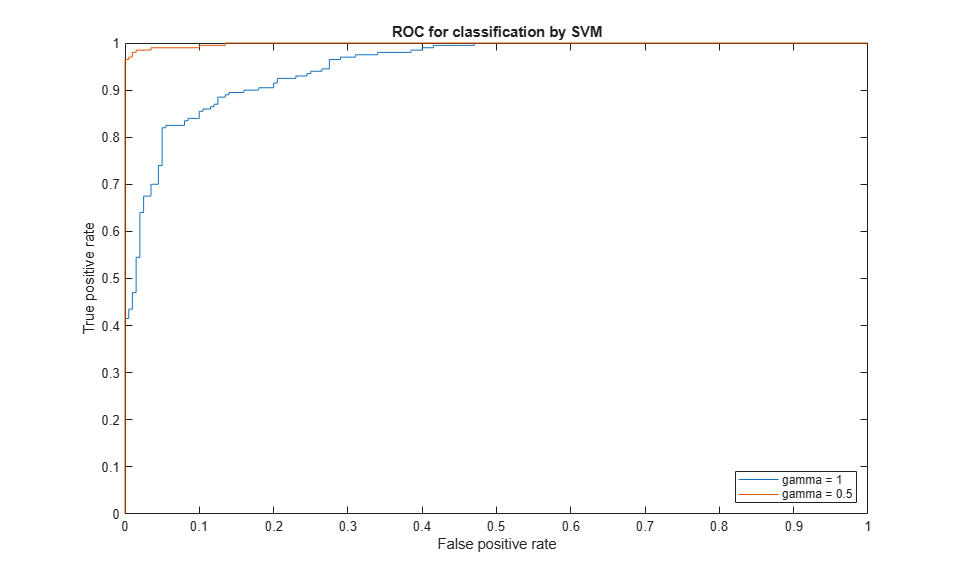The kernel function with the gamma parameter set to 0.5 gives better in-sample results.

Compare the AUC measures.

```auc1 auc2 ```
```auc1 = 0.9518 auc2 = 0.9985 ```

The area under the curve for gamma set to 0.5 is higher than that for gamma set to 1. This also confirms that gamma parameter value of 0.5 produces better results. For visual comparison of the classification performance with these two gamma parameter values, see Train SVM Classifier Using Custom Kernel.

`load fisheriris`

The column vector, `species`, consists of iris flowers of three different species: setosa, versicolor, virginica. The double matrix `meas` consists of four types of measurements on the flowers: sepal length, sepal width, petal length, and petal width. All measures are in centimeters.

Train a classification tree using the sepal length and width as the predictor variables. It is a good practice to specify the class names.

```Model = fitctree(meas(:,1:2),species, ... 'ClassNames',{'setosa','versicolor','virginica'});```

Predict the class labels and scores for the species based on the tree `Model`.

`[~,score] = resubPredict(Model);`

The scores are the posterior probabilities that an observation (a row in the data matrix) belongs to a class. The columns of `score` correspond to the classes specified by `'ClassNames'`. So, the first column corresponds to setosa, the second corresponds to versicolor, and the third column corresponds to virginica.

Compute the ROC curve for the predictions that an observation belongs to versicolor, given the true class labels `species`. Also compute the optimal operating point and y values for negative subclasses. Return the names of the negative classes.

Because this is a multiclass problem, you cannot merely supply `score(:,2)` as input to `perfcurve`. Doing so would not give `perfcurve` enough information about the scores for the two negative classes (setosa and virginica). This problem is unlike a binary classification problem, where knowing the scores of one class is enough to determine the scores of the other class. Therefore, you must supply `perfcurve` with a function that factors in the scores of the two negative classes. One such function is $score\left(:,2\right)-\mathrm{max}\left(score\left(:,1\right),score\left(:,3\right)\right)$.

```diffscore = score(:,2) - max(score(:,1),score(:,3)); [X,Y,T,~,OPTROCPT,suby,subnames] = perfcurve(species,diffscore,'versicolor');```

`X`, by default, is the false positive rate (fallout or 1-specificity) and `Y`, by default, is the true positive rate (recall or sensitivity). The positive class label is `versicolor`. Because a negative class is not defined, `perfcurve` assumes that the observations that do not belong to the positive class are in one class. The function accepts it as the negative class.

`OPTROCPT`
```OPTROCPT = 1×2 0.1000 0.8000 ```
`suby`
```suby = 12×2 0 0 0.1800 0.1800 0.4800 0.4800 0.5800 0.5800 0.6200 0.6200 0.8000 0.8000 0.8800 0.8800 0.9200 0.9200 0.9600 0.9600 0.9800 0.9800 ⋮ ```
`subnames`
```subnames = 1x2 cell {'setosa'} {'virginica'} ```

Plot the ROC curve and the optimal operating point on the ROC curve.

```plot(X,Y) hold on plot(OPTROCPT(1),OPTROCPT(2),'ro') xlabel('False positive rate') ylabel('True positive rate') title('ROC Curve for Classification by Classification Trees') hold off```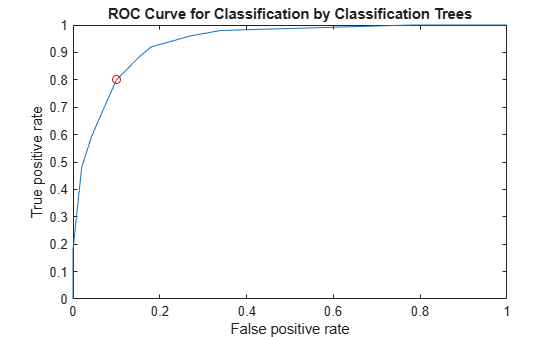Find the threshold that corresponds to the optimal operating point.

`T((X==OPTROCPT(1))&(Y==OPTROCPT(2)))`
```ans = 0.2857 ```

Specify `virginica` as the negative class and compute and plot the ROC curve for `versicolor`.

Again, you must supply `perfcurve` with a function that factors in the scores of the negative class. An example of a function to use is $score\left(:,2\right)-score\left(:,3\right)$.

```diffscore = score(:,2) - score(:,3); [X,Y,~,~,OPTROCPT] = perfcurve(species,diffscore,'versicolor', ... 'negClass','virginica'); OPTROCPT```
```OPTROCPT = 1×2 0.1800 0.8200 ```
```figure, plot(X,Y) hold on plot(OPTROCPT(1),OPTROCPT(2),'ro') xlabel('False positive rate') ylabel('True positive rate') title('ROC Curve for Classification by Classification Trees') hold off```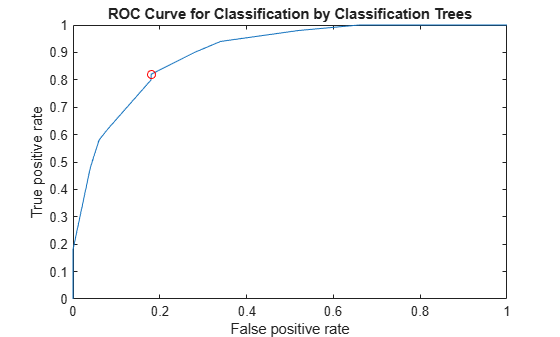`load fisheriris`

The column vector `species` consists of iris flowers of three different species: setosa, versicolor, virginica. The double matrix `meas` consists of four types of measurements on the flowers: sepal length, sepal width, petal length, and petal width. All measures are in centimeters.

Use only the first two features as predictor variables. Define a binary problem by using only the measurements that correspond to the versicolor and virginica species.

`pred = meas(51:end,1:2);`

Define the binary response variable.

`resp = (1:100)'>50; % Versicolor = 0, virginica = 1`

Fit a logistic regression model.

`mdl = fitglm(pred,resp,'Distribution','binomial','Link','logit');`

Compute the pointwise confidence intervals on the true positive rate (TPR) by vertical averaging (VA) and sampling using bootstrap.

```[X,Y,T] = perfcurve(species(51:end,:),mdl.Fitted.Probability,... 'virginica','NBoot',1000,'XVals',[0:0.05:1]);```

`'NBoot',1000` sets the number of bootstrap replicas to 1000. `'XVals','All'` prompts `perfcurve` to return `X`, `Y`, and `T` values for all scores, and average the `Y` values (true positive rate) at all `X` values (false positive rate) using vertical averaging. If you do not specify `XVals`, then `perfcurve` computes the confidence bounds using threshold averaging by default.

Plot the pointwise confidence intervals.

```errorbar(X,Y(:,1),Y(:,1)-Y(:,2),Y(:,3)-Y(:,1)); xlim([-0.02,1.02]); ylim([-0.02,1.02]); xlabel('False positive rate') ylabel('True positive rate') title('ROC Curve with Pointwise Confidence Bounds') legend('PCBwVA','Location','Best')```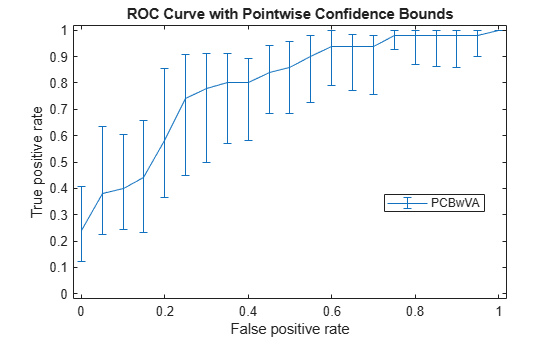It might not always be possible to control the false positive rate (FPR, the `X` value in this example). So you might want to compute the pointwise confidence intervals on true positive rates (TPR) by threshold averaging.

```[X1,Y1,T1] = perfcurve(species(51:end,:),mdl.Fitted.Probability,... 'virginica','NBoot',1000);```

If you set `'TVals'` to `'All'`, or if you do not specify `'TVals'` or `'Xvals'`, then `perfcurve` returns `X`, `Y`, and `T` values for all scores and computes pointwise confidence bounds for `X` and `Y` using threshold averaging.

Plot the confidence bounds.

```figure() errorbar(X1(:,1),Y1(:,1),Y1(:,1)-Y1(:,2),Y1(:,3)-Y1(:,1)); xlim([-0.02,1.02]); ylim([-0.02,1.02]); xlabel('False positive rate') ylabel('True positive rate') title('ROC Curve with Pointwise Confidence Bounds') legend('PCBwTA','Location','Best')```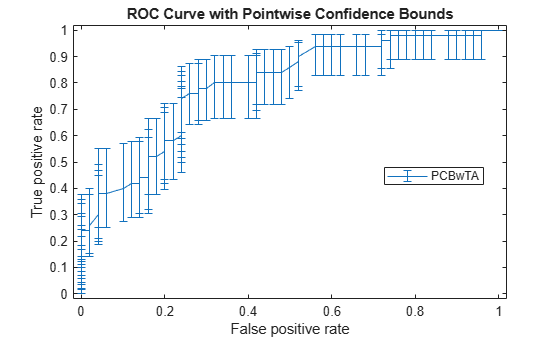Specify the threshold values to fix and compute the ROC curve. Then plot the curve.

```[X1,Y1,T1] = perfcurve(species(51:end,:),mdl.Fitted.Probability,... 'virginica','NBoot',1000,'TVals',0:0.05:1); figure() errorbar(X1(:,1),Y1(:,1),Y1(:,1)-Y1(:,2),Y1(:,3)-Y1(:,1)); xlim([-0.02,1.02]); ylim([-0.02,1.02]); xlabel('False positive rate') ylabel('True positive rate') title('ROC Curve with Pointwise Confidence Bounds') legend('PCBwTA','Location','Best')```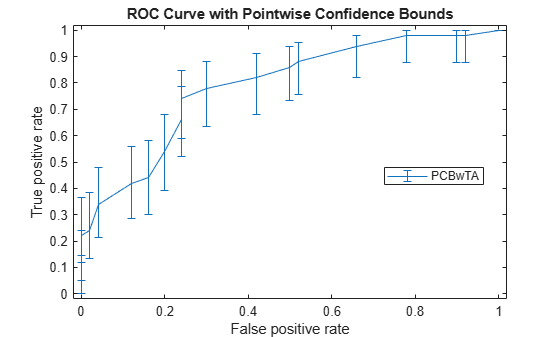## Input Arguments

collapse all

True class labels, specified as a numeric vector, logical vector, character matrix, string array, cell array of character vectors, or categorical array. For more information, see Grouping Variables.

Example: `{'hi','mid','hi','low',...,'mid'}`

Example: `['H','M','H','L',...,'M']`

Data Types: `single` | `double` | `logical` | `char` | `string` | `cell` | `categorical`

Scores returned by a classifier for some sample data, specified as a vector of floating points. `scores` must have the same number of elements as `labels`.

Data Types: `single` | `double`

Positive class label, specified as a numeric scalar, logical scalar, character vector, string scalar, cell containing a character vector, or categorical scalar. The positive class must be a member of the input labels. The value of `posclass` that you can specify depends on the value of `labels`.

`labels` value`posclass` value
Numeric vectorNumeric scalar
Logical vectorLogical scalar
Character matrixCharacter vector
String arrayString scalar
Cell array of character vectorsCharacter vector or cell containing character vector
Categorical vector Categorical scalar

For example, in a cancer diagnosis problem, if a malignant tumor is the positive class, then specify `posclass` as `'malignant'`.

Data Types: `single` | `double` | `logical` | `char` | `string` | `cell` | `categorical`

### Name-Value Pair Arguments

Specify optional comma-separated pairs of `Name,Value` arguments. `Name` is the argument name and `Value` is the corresponding value. `Name` must appear inside quotes. You can specify several name and value pair arguments in any order as `Name1,Value1,...,NameN,ValueN`.

Example: `'NegClass','versicolor','XCrit','fn','NBoot',1000,'BootType','per'` specifies the species versicolor as the negative class, the criterion for the X-coordinate as false negative, the number of bootstrap samples as 1000. It also specifies that the pointwise confidence bounds are computed using the percentile method.

List of negative classes, specified as the comma-separated pair consisting of `'NegClass'`, and a numeric array, a categorical array, a string array, or a cell array of character vectors. By default, `perfcurve` sets `NegClass` to `'all'` and considers all nonpositive classes found in the input array of labels to be negative.

If `NegClass` is a subset of the classes found in the input array of labels, then `perfcurve` discards the instances with labels that do not belong to either positive or negative classes.

Example: `'NegClass',{'versicolor','setosa'}`

Data Types: `single` | `double` | `categorical` | `char` | `string` | `cell`

Criterion to compute for `X`, specified as the comma-separated pair consisting of `'XCrit'` and one of the following.

CriterionDescription
`tp`Number of true positive instances
`fn`Number of false negative instances.
`fp`Number of false positive instances.
`tn`Number of true negative instances.
`tp+fp`Sum of true positive and false positive instances.
`rpp`Rate of positive predictions.
```rpp = (tp+fp)/(tp+fn+fp+tn)```
`rnp`Rate of negative predictions.
```rnp = (tn+fn)/(tp+fn+fp+tn)```
`accu`Accuracy.
`accu = (tp+tn)/(tp+fn+fp+tn)`
`tpr`, or `sens`, or `reca`True positive rate, or sensitivity, or recall.
```tpr= sens = reca = tp/(tp+fn)```
`fnr`, or `miss` False negative rate, or miss.
```fnr = miss = fn/(tp+fn)```
`fpr`, or `fall`False positive rate, or fallout, or 1 – specificity.
`fpr = fall = fp/(tn+fp)`
`tnr`, or `spec`True negative rate, or specificity.
```tnr = spec = tn/(tn+fp)```
`ppv`, or `prec`Positive predictive value, or precision.
```ppv = prec = tp/(tp+fp)```
`npv`Negative predictive value.
`npv = tn/(tn+fn)`
`ecost`Expected cost.
```ecost = (tp*Cost(P|P)+fn*Cost(N|P)+fp* Cost(P|N)+tn*Cost(N|N))/(tp+fn+fp+tn)```
Custom criterionA custom-defined function with the input arguments `(C,scale,cost)`, where `C` is a 2-by-2 confusion matrix, `scale` is a 2-by-1 array of class scales, and `cost` is a 2-by-2 misclassification cost matrix.

### Caution

Some of these criteria return `NaN` values at one of the two special thresholds, `'reject all'` and ```'accept all'```.

Example: `'XCrit','ecost'`

Criterion to compute for `Y`, specified as the comma-separated pair consisting of `'YCrit'` and one of the same criteria options as for `X`. This criterion does not have to be a monotone function of the positive class score.

Example: `'YCrit','ecost'`

Values for the `X` criterion, specified as the comma-separated pair consisting of `'XVals'` and a numeric array.

• If you specify `XVals`, then `perfcurve` computes `X` and `Y` and the pointwise confidence bounds for `Y` (when applicable) only for the specified `XVals`.

• If you do not specify `XVals`, then `perfcurve`, computes `X` and `Y` and the values for all scores by default.

### Note

You cannot set `XVals` and `TVals` at the same time.

Example: `'XVals',[0:0.05:1]`

Data Types: `single` | `double` | `char` | `string`

Thresholds for the positive class score, specified as the comma-separated pair consisting of `'TVals'` and either `'all'` or a numeric array.

• If `TVals` is set to `'all'` or not specified, and `XVals` is not specified, then `perfcurve` returns `X`, `Y`, and `T` values for all scores and computes pointwise confidence bounds for `X` and `Y` using threshold averaging.

• If `TVals` is set to a numeric array, then `perfcurve` returns `X`, `Y`, and `T` values for the specified thresholds and computes pointwise confidence bounds for `X` and `Y` at these thresholds using threshold averaging.

### Note

You cannot set `XVals` and `TVals` at the same time.

Example: `'TVals',[0:0.05:1]`

Data Types: `single` | `double` | `char` | `string`

Indicator to use the nearest values in the data instead of the specified numeric `XVals` or `TVals`, specified as the comma-separated pair consisting of `'UseNearest'` and either `'on'` or `'off'`.

• If you specify numeric `XVals` and set `UseNearest` to `'on'`, then `perfcurve` returns the nearest unique `X` values found in the data, and it returns the corresponding values of `Y` and `T`.

• If you specify numeric `XVals` and set `UseNearest` to `'off'`, then `perfcurve` returns the sorted `XVals`.

• If you compute confidence bounds by cross validation or bootstrap, then this parameter is always `'off'`.

Example: `'UseNearest','off'`

`perfcurve` method for processing `NaN` scores, specified as the comma-separated pair consisting of `'ProcessNaN'` and `'ignore'` or `'addtofalse'`.

• If `ProcessNaN` is `'ignore'`, then `perfcurve` removes observations with `NaN` scores from the data.

• If `ProcessNaN` is `'addtofalse'`, then `perfcurve` adds instances with `NaN` scores to false classification counts in the respective class. That is, `perfcurve` always counts instances from the positive class as false negative (FN), and it always counts instances from the negative class as false positive (FP).

Example: `'ProcessNaN','addtofalse'`

Prior probabilities for positive and negative classes, specified as the comma-separated pair consisting of `'Prior'` and `'empirical'`, `'uniform'`, or an array with two elements.

If `Prior` is `'empirical'`, then `perfcurve` derives prior probabilities from class frequencies.

If `Prior` is `'uniform'` , then `perfcurve` sets all prior probabilities to be equal.

Example: `'Prior',[0.3,0.7]`

Data Types: `single` | `double` | `char` | `string`

Misclassification costs, specified as the comma-separated pair consisting of `'Cost'` and a 2-by-2 matrix, containing `[Cost(P|P),Cost(N|P);Cost(P|N),Cost(N|N)]`.

`Cost(N|P)` is the cost of misclassifying a positive class as a negative class. `Cost(P|N)` is the cost of misclassifying a negative class as a positive class. Usually, `Cost(P|P)` = 0 and `Cost(N|N)` = 0, but `perfcurve` allows you to specify nonzero costs for correct classification as well.

Example: `'Cost',[0 0.7;0.3 0]`

Data Types: `single` | `double`

Significance level for the confidence bounds, specified as the comma-separated pair consisting of `'Alpha'` and a scalar value in the range 0 through 1. `perfcurve` computes 100*(1 – α) percent pointwise confidence bounds for `X`, `Y`, `T`, and `AUC` for a confidence level of 1 – α.

Example: `'Alpha',0.01` specifies 99% confidence bounds

Data Types: `single` | `double`

Observation weights, specified as the comma-separated pair consisting of `'Weights'` and a vector of nonnegative scalar values. This vector must have as many elements as `scores` or `labels` do.

If `scores` and `labels` are in cell arrays and you need to supply `Weights`, the weights must be in a cell array as well. In this case, every element in `Weights` must be a numeric vector with as many elements as the corresponding element in `scores`. For example, `numel(weights{1}) == numel(scores{1})`.

When `perfcurve` computes the `X`, `Y` and `T` or confidence bounds using cross-validation, it uses these observation weights instead of observation counts.

When `perfcurve` computes confidence bounds using bootstrap, it samples N out of N observations with replacement, using these weights as multinomial sampling probabilities.

The default is a vector of 1s or a cell array in which each element is a vector of 1s.

Data Types: `single` | `double` | `cell`

Number of bootstrap replicas for computation of confidence bounds, specified as the comma-separated pair consisting of `'NBoot'` and a positive integer. The default value 0 means the confidence bounds are not computed.

If `labels` and `scores` are cell arrays, this parameter must be 0 because `perfcurve` can use either cross-validation or bootstrap to compute confidence bounds.

Example: `'NBoot',500`

Data Types: `single` | `double`

Confidence interval type for `bootci` to use to compute confidence bounds, specified as the comma-separated pair consisting of `'BootType'` and one of the following:

• `'bca'` — Bias corrected and accelerated percentile method

• `'norm` or `'normal'` — Normal approximated interval with bootstrapped bias and standard error

• `'per'` or `'percentile'` — Percentile method

• `'cper'` or `'corrected percentile'` — Bias corrected percentile method

• `'stud'` or `'student'` — Studentized confidence interval

Example: `'BootType','cper'`

Optional input arguments for `bootci` to compute confidence bounds, specified as the comma-separated pair consisting of `'BootArg'` and `{'Nbootstd',nbootstd}` or `{'Stderr',stderr}`, name-value pair arguments of `bootci`.

When you compute the studentized bootstrap confidence intervals (`'BootType'` is `'student'`), you can additionally specify `'Nbootstd'` or `'Stderr'`, name-value pair arguments of `bootci`, by using `'BootArg'`.

• `'BootArg',{'Nbootstd',nbootstd}` estimates the standard error of the bootstrap statistics using bootstrap with `nbootstd` data samples. `nbootstd` is a positive integer and its default is 100.

• `'BootArg',{'Stderr',stderr}` evaluates the standard error of the bootstrap statistics by a user-defined function `stderr` that takes `[1:numel(scores)]'` as an input argument. `stderr` is a function handle.

Example: `'BootArg',{'Nbootstd',nbootstd}`

Data Types: `cell`

Options for controlling the computation of confidence intervals, specified as the comma-separated pair consisting of `'Options'` and a structure array returned by `statset`. These options require Parallel Computing Toolbox™. `perfcurve` uses this argument for computing pointwise confidence bounds only. To compute these bounds, you must pass cell arrays for `labels` and `scores` or set `NBoot` to a positive integer.

This table summarizes the available options.

OptionDescription
`'UseParallel'`
• `false` — Serial computation (default).

• `true` — Parallel computation. You need Parallel Computing Toolbox for this option to work.

`'UseSubstreams'`
• `false` — Do not use a separate substream for each iteration (default).

• `true` — Use a separate substream for each iteration to compute in parallel in a reproducible fashion. To compute reproducibly, set `Streams` to a type allowing substreams: `'mlfg6331_64'` or `'mrg32k3a'`.

`'Streams'`

A `RandStream` object, or a cell array of such objects. If you specify `Streams`, use a single object, except when:

• `UseParallel` is `true`.

• `UseSubstreams` is `false`.

In that case, use a cell array of the same size as the parallel pool. If a parallel pool is not open, then `Streams` must supply a single random number stream.

If `'UseParallel'` is `true` and `'UseSubstreams'` is `false`, then the length of `'Streams'` must equal the number of workers used by `perfcurve`. If a parallel pool is already open, then the length of `'Streams'` is the size of the parallel pool. If a parallel pool is not already open, then MATLAB® might open a pool for you, depending on your installation and preferences. To ensure more predictable results, use `parpool` and explicitly create a parallel pool before invoking `perfcurve` and setting `'Options',statset('UseParallel',true)`.

Example: `'Options',statset('UseParallel',true)`

Data Types: `struct`

## Output Arguments

collapse all

x-coordinates for the performance curve, returned as a vector or an m-by-3 matrix. By default, `X` values are the false positive rate, FPR (fallout or 1 – specificity). To change `X`, use the `XCrit` name-value pair argument.

• If `perfcurve` does not compute the pointwise confidence bounds, or if it computes them using vertical averaging, then `X` is a vector.

• If `perfcurve` computes the confidence bounds using threshold averaging, then `X` is an m-by-3 matrix, where m is the number of fixed threshold values. The first column of `X` contains the mean value. The second and third columns contain the lower bound and the upper bound, respectively, of the pointwise confidence bounds.

y-coordinates for the performance curve, returned as a vector or an m-by-3 matrix. By default, `Y` values are the true positive rate, TPR (recall or sensitivity). To change `Y`, use `YCrit` name-value pair argument.

• If `perfcurve` does not compute the pointwise confidence bounds, then `Y` is a vector.

• If `perfcurve` computes the confidence bounds, then `Y` is an m-by-3 matrix, where m is the number of fixed `X` values or thresholds (`T` values). The first column of `Y` contains the mean value. The second and third columns contain the lower bound and the upper bound, respectively, of the pointwise confidence bounds.

Thresholds on classifier scores for the computed values of `X` and `Y`, returned as a vector or m-by-3 matrix.

• If `perfcurve` does not compute the pointwise confidence bounds, or computes them using threshold averaging, then `T` is a vector.

• If `perfcurve` computes the confidence bounds using vertical averaging, `T` is an m-by-3 matrix, where m is the number of fixed `X` values. The first column of `T` contains the mean value. The second and third columns contain the lower bound, and the upper bound, respectively, of the pointwise confidence bounds.

For each threshold, `TP` is the count of true positive observations with scores greater than or equal to this threshold, and `FP` is the count of false positive observations with scores greater than or equal to this threshold. `perfcurve` defines negative counts, `TN` and `FN`, in a similar way. The function then sorts the thresholds in the descending order that corresponds to the ascending order of positive counts.

For the m distinct thresholds found in the array of scores, `perfcurve` returns the `X`, `Y` and `T` arrays with m + 1 rows. `perfcurve` sets elements `T(2:m+1)` to the distinct thresholds, and `T(1)` replicates `T(2)`. By convention, `T(1)` represents the highest ```'reject all'``` threshold, and `perfcurve` computes the corresponding values of `X` and `Y` for ```TP = 0``` and `FP = 0`. The `T(end)` value is the lowest `'accept all'` threshold for which ```TN = 0``` and `FN = 0`.

Area under the curve (`AUC`) for the computed values of `X` and `Y`, returned as a scalar value or a 3-by-1 vector.

• If `perfcurve` does not compute the pointwise confidence bounds, `AUC` is a scalar value.

• If `perfcurve` computes the confidence bounds using vertical averaging, `AUC` is a 3-by-1 vector. The first column of `AUC` contains the mean value. The second and third columns contain the lower bound and the upper bound, respectively, of the confidence bound.

For a perfect classifier, AUC = 1. For a classifier that randomly assigns observations to classes, AUC = 0.5.

If you set `XVals` to `'all'` (default), then `perfcurve` computes `AUC` using the returned `X` and `Y` values.

If `XVals` is a numeric array, then `perfcurve` computes `AUC` using `X` and `Y` values from all distinct scores in the interval, which are specified by the smallest and largest elements of `XVals`. More precisely, `perfcurve` finds `X` values for all distinct thresholds as if `XVals` were set to `'all'`, and then uses a subset of these (with corresponding `Y` values) between `min(XVals)` and `max(XVals)` to compute `AUC`.

`perfcurve` uses trapezoidal approximation to estimate the area. If the first or last value of `X` or `Y` are `NaN`s, then `perfcurve` removes them to allow calculation of `AUC`. This takes care of criteria that produce `NaN`s for the special `'reject all'` or ```'accept all'``` thresholds, for example, positive predictive value (PPV) or negative predictive value (NPV).

Optimal operating point of the ROC curve, returned as a 1-by-2 array with false positive rate (FPR) and true positive rate (TPR) values for the optimal ROC operating point.

`perfcurve` computes `OPTROCPT` for the standard ROC curve only, and sets to `NaN`s otherwise. To obtain the optimal operating point for the ROC curve, `perfcurve` first finds the slope, S, using

`$S=\frac{\text{Cost}\left(P|N\right)-\text{Cost}\left(N|N\right)}{\text{Cost}\left(N|P\right)-\text{Cost}\left(P|P\right)}*\frac{N}{P}$`

• Cost(N|P) is the cost of misclassifying a positive class as a negative class. Cost(P|N) is the cost of misclassifying a negative class as a positive class.

• P = TP + FN and N = TN + FP. They are the total instance counts in the positive and negative class, respectively.

`perfcurve` then finds the optimal operating point by moving the straight line with slope S from the upper left corner of the ROC plot (`FPR = 0`, ```TPR = 1```) down and to the right, until it intersects the ROC curve.

Values for negative subclasses, returned as an array.

• If you specify only one negative class, then `SUBY` is identical to `Y`.

• If you specify k negative classes, then `SUBY` is a matrix of size m-by-k, where m is the number of returned values for `X` and `Y`, and k is the number of negative classes. `perfcurve` computes `Y` values by summing counts over all negative classes.

`SUBY` gives values of the `Y` criterion for each negative class separately. For each negative class, `perfcurve` places a new column in `SUBY` and fills it with `Y` values for true negative (TN) and false positive (FP) counted just for this class.

Negative class names, returned as a cell array.

• If you provide an input array of negative class names, , `NegClass`, then `perfcurve` copies names into `SUBYNAMES`.

• If you do not provide `NegClass`, then `perfcurve` extracts `SUBYNAMES` from the input labels. The order of `SUBYNAMES` is the same as the order of columns in `SUBY`. That is, `SUBY(:,1)` is for negative class `SUBYNAMES{1}`, `SUBY(:,2)` is for negative class `SUBYNAMES{2}`, and so on.

## Algorithms

collapse all

### Pointwise Confidence Bounds

If you supply cell arrays for `labels` and `scores`, or if you set `NBoot` to a positive integer, then `perfcurve` returns pointwise confidence bounds for `X`,`Y`,`T`, and `AUC`. You cannot supply cell arrays for `labels` and `scores` and set `NBoot` to a positive integer at the same time.

`perfcurve` resamples data to compute confidence bounds using either cross validation or bootstrap.

• Cross-validation — If you supply cell arrays for `labels` and `scores`, then `perfcurve` uses cross-validation and treats elements in the cell arrays as cross-validation folds. `labels` can be a cell array of numeric vectors, logical vectors, character matrices, cell arrays of character vectors, or categorical vectors. All elements in `labels` must have the same type. `scores` can be a cell array of numeric vectors. The cell arrays for `labels` and `scores` must have the same number of elements. The number of labels in cell j of `labels` must be equal to the number of scores in cell j of `scores` for any j in the range from 1 to the number of elements in `scores`.

• Bootstrap — If you set `NBoot` to a positive integer n, `perfcurve` generates n bootstrap replicas to compute pointwise confidence bounds. If you use `XCrit` or `YCrit` to set the criterion for `X` or `Y` to an anonymous function, `perfcurve` can compute confidence bounds only using bootstrap.

`perfcurve` estimates the confidence bounds using one of two methods:

• Vertical averaging (VA) — `perfcurve` estimates confidence bounds on `Y` and `T` at fixed values of `X`. That is, `perfcurve` takes samples of the ROC curves for fixed `X` values, averages the corresponding `Y` and `T` values, and computes the standard errors. You can use the `XVals` name-value pair argument to fix the `X` values for computing confidence bounds. If you do not specify `XVals`, then `perfcurve` computes the confidence bounds at all `X` values.

• Threshold averaging (TA) — `perfcurve` takes samples of the ROC curves at fixed thresholds `T` for the positive class score, averages the corresponding `X` and `Y` values, and estimates the confidence bounds. You can use the `TVals` name-value pair argument to use this method for computing confidence bounds. If you set `TVals` to `'all'` or do not specify `TVals` or `XVals`, then `perfcurve` returns `X`, `Y`, and `T` values for all scores and computes pointwise confidence bounds for `Y` and `X` using threshold averaging.

When you compute the confidence bounds, `Y` is an m-by-3 array, where m is the number of fixed `X` values or thresholds (`T` values). The first column of `Y` contains the mean value. The second and third columns contain the lower bound and the upper bound, respectively, of the pointwise confidence bounds. `AUC` is a row vector with three elements, following the same convention. If `perfcurve` computes the confidence bounds using VA, then `T` is an m-by-3 matrix, and `X` is a column vector. If `perfcurve` uses TA, then `X` is an m-by-3 matrix and `T` is a column-vector.

`perfcurve` returns pointwise confidence bounds. It does not return a simultaneous confidence band for the entire curve.

 T. Fawcett. “ROC Graphs: Notes and Practical Considerations for Researchers”, 2004.

 Zweig, M., and G. Campbell. “Receiver-Operating Characteristic (ROC) Plots: A Fundamental Evaluation Tool in Clinical Medicine.” Clin. Chem. 1993, 39/4, pp. 561–577 .

 Davis, J., and M. Goadrich. “The Relationship Between Precision-Recall and ROC Curves.” Proceedings of ICML ’06, 2006, pp. 233–240.

 Moskowitz, C., and M. Pepe. “Quantifying and comparing the predictive accuracy of continuous prognostic factors for binary outcomes.” Biostatistics, 2004, 5, pp. 113–127.

 Huang, Y., M. Pepe, and Z. Feng. “Evaluating the Predictiveness of a Continuous Marker.” U. Washington Biostatistics Paper Series, 2006, 250–261.

 Briggs, W., and R. Zaretzki. “The Skill Plot: A Graphical Technique for Evaluating Continuous Diagnostic Tests.” Biometrics, 2008, 63, pp. 250 – 261.

 R. Bettinger. “Cost-Sensitive Classifier Selection Using the ROC Convex Hull Method.” SAS Institute.